```
``````

```
The Internal Structure of Crystals   (Part Two)
```

```
back to homepage

The symmetry of regular 2-D arrays of repeated motifs (continued)

In previous Part we have discussed the five fundamental 2-D lattice types and their point symmetry. We also discussed the ten fundamental 2-D point symmetries of motifs, and we began to discuss the insertion of motifs in those lattices, in order to obtain two-dimensional arrays of repeated motifs, representing the internal structure of imaginary two-dimensional crystals. The aim of all this is to gain insight in the development of the shapes of real, i.e. 3-D crystals.
We will now continue the discussion of the proper insertion of motifs into the five 2-D lattices (nets), resulting in the 17 possible Plane Groups, i.e. we're going to take into account not only point symmetries but also translational symmetries (simple translation and glide lines). The paterns so obtained are periodic arrays. There are several ways to indicate a unit in it that is repeated periodically throughout the structure. This is the unit mesh. We prefer to choose a unit mesh such that its corners coincide with nodes of the net. If such a unit mesh only contains nodes at its corners, it is called a primitive unit mesh (P), if it also has a node in its center, it is called a centered unit mesh(C).

Obliquenet
The Oblique Net (2-D Oblique Lattice) has point symmetry 2. It does not support mirror lines. The only symmetry element it supports is a 2-fold rotation axis. So we are confident that motifs, possessing a point symmetry of  2,  i.e. possessing a 2-fold rotation axis as the only symmetry element, can be inserted into the Oblique Net. See Figure 1.Figure 1.  Placing 2-D motifs with point symmetry 2 into an oblique 2-D lattice yields a pattern that represents the Plane Group P2. Each motif consists of two motif units (commas). As in all other such Figures (coming up below), the pattern must be imagined to be extended indefinitely over two-dimensional space.

```
```
In the above Figure the motif consists of two motif units (commas), in such a way that the motif is going to possess a two-fold rotation axis. And this is the motif in the strict sense (We can write "motif s.str."). But in fact the surroundings of such a motif also belong to that motif. Only then it effects a complete tiling of the 2-D plane. These surroundings are indicated in the next Figure. A motif s.str. + surroundings, such that together they form a unit that, when repeated, tiles the plane, is a motif in the broader sense (motif s.l.).Figure 1a.  Motif s.str. and motif s.l.

As a unit mesh (which is the two-dimensional analogue to the unit cell in three dimensions) we could choose the motif s.l., but it is preferable to choose the unit mesh such that it has lattice nodes at its corners. This unit mesh is the non-empty building block of the 2-D crystal. Periodic repeat of this building block yields the whole structure. Because the (chosen) unit mesh has no node in its center, it is a primitive unit mesh, indicated by the symbol P. (Other choices of unit mesh are still possible, but things will be more convenient when the edges of the unit mesh are parallel to the crystallographic directions x and y of the net (See for these directions Figure 3 of Part One)).Figure 1b.  A possible choice of unit mesh (yellow). The content of this mesh consists of two half motifs, together making up just one motif (consisting of two motif units related to each other by a 2-fold rotation axis). The point symmetry of this unit mesh is 2.

```
```
If the Plane Group P2 is given, then we can deduce its point symmetry, by eliminating every translational aspect. We, as it were, slide the whole structure together, we compress it, telescope it inward, till every translation is brought back to zero translation. We then end up with a single object (a figure). The point symmetry of this figure then is the point symmetry of the periodic pattern of motifs. See Figure 1c.Figure 1c.  Figure, emerging when all translations are eliminated. This figure has a point symmetry 2, and is the translation-free residue of the (total symmetry of the) pattern of Figure 1, i.e. it represents the point symmetry of that pattern.

```
```
In graphic illustrations of Plane Groups one normally only depicts the symmetry content without drawing any motif. Indeed, from that symmetry content the symmetry of the motif can be deduced. In the next Figure we give the total symmetry content of the Plane Group P2.Figure 1d. The total symmetry content of the Plane Group P2 :   simple translation (two translation vectors), 2-fold rotation axes.

```
```
The second type of motif we could insert in an oblique net is a motif with a lesser degree of symmetry than the one above. This boils down to a motif with no symmetry at all. See Figure 2.Figure 2.  Placing 2-D motifs with point symmetry 1 into an oblique 2-D lattice yields a pattern that represents the Plane Group P1.

```
```Figure 2a.  A unit mesh choice for the pattern of Figure 2 is given in yellow. This unit mesh, not containing a motif in its center, is primitive and is denoted by the symbol P. Its point symmetry is 1.

```
```
The motif s.str. and motif s.l. is given in the next Figure.Figure 2b.  Repeated motif with point symmetry 1 in the pattern of Figure 2.
It is, in the present case, at the same time the figure that emerges when all translations are eliminated. Its point symmetry is
1 and as such gives the point symmetry of the pattern of Figure 2, i.e. the translation-free residue of the pattern's (total) symmetry.

```
```
The total symmetry content of Plane Group P1 is given in the next Figure.Figure 2c.  Total symmetry content of Plane Group P1.
The only symmetry present is : simple translation (in two directions).

```
```
We now have derived from the Oblique Net the two Point Groups associated with that net (lattice), namely 2 and 1. They are the two Crystal Classes of the 2-D Oblique Crystal System. Each Class supports a Plane Group :   P2 and P1 respectively.
```
```
Square Net
The Square Net (2-D Square Lattice) has point symmetry 4mm, so it does support 4-fold rotation and two types of mirror line. Consequently it surely can accommodate the insertion of a motif that has 4mm symmetry. This results in a periodic pattern that represents the Plane Group P4mm. See Figure 3.Figure 3.  Placing 2-D motifs with point symmetry 4mm into a square 2-D lattice yields a pattern that represents the Plane Group P4mm.

```
```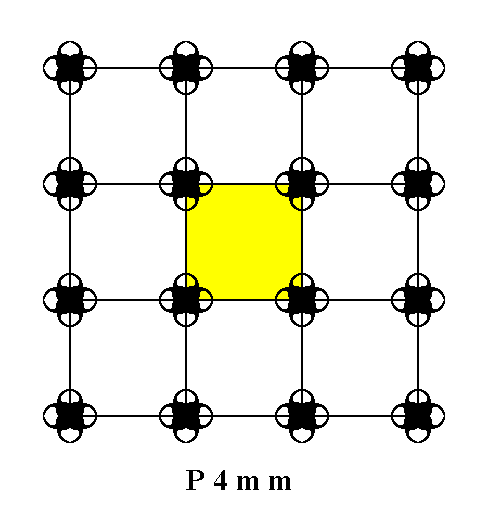Figure 3a.  A unit mesh choice is given in yellow. This unit mesh contains four 1/4 motifs, which is equivalent to it containing precisely one motif. This unit mesh, not containing a motif in its center, is primitive and is denoted by the symbol P. Its point symmetry is 4mm .

```
```
The motif s.str. and motif s.l. are depicted in the next Figure.Figure 3b.  Repeated motif with point symmetry 4mm in the pattern of Figure 3.
This is, in the present case, also the figure that emerges when all translations are eliminated. Its point symmetry is
4mm and as such gives the point symmetry of the pattern of Figure 3.

```
```
Besides simple translation the periodic pattern representing the Plane Group P4mm contains glide lines. The next Figure depicts one of these glide lines.Figure 3c.  One of the possible glide lines in the pattern of Figure 3.

```
```
The total symmetry content of the Plane Group P4mm consists of simple translations, glide lines, 4-fold rotations and two types of mirror line. See Figure 3d.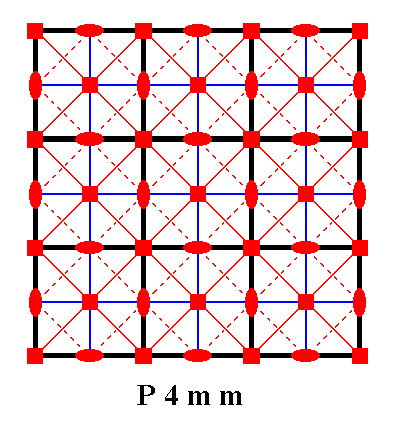Figure 3d.  Total symmetry content of the Plane Group P4mm.
All continuous lines, including those that represent (the lines connecting the nodes of) the net, are mirror lines. There are two types of them, diagonal and non-diagonal. Dashed lines signify glide lines, small solid ellipses signify 2-fold rotation axes perpendicular to the plane of the drawing, and small solid squares signify 4-fold rotation axes perpendicular to the plane of the drawing.

```
```
There are still several other motifs (having different symmetries) that can be accommodated in a Square Net. These motifs have lower symmetry than the one discussed above. The resulting periodic pattern expresses the motif's lower symmetry.
One of these periodic patterns is especially instructive for a general understanding of two-dimensional periodic arrays. i.e. for an understanding of two-dimensional crystals, and with it an understanding of three-dimensional crystals. It is the Plane group P4gm. So we will dwell a little longer upon one of the patterns representing this Plane Group.Figure 4.  A periodic pattern of motifs based on a 2-D square lattice (net). This particular pattern represents the Plane Group P4gm. It must be imagined to extend indefinitely over the 2-D plane.

```
```Figure 4a.  A unit mesh choice for the above pattern is given in yellow.
The pattern consists of some element (i.e. a proper motif, already present in, but yet to be found and indicated) which is repeated by translation vector
a  in the direction  x , and by translation vector  b  in direction  y.  In the present case the length of  a  is equal to that of  b.

```
```
When we eliminate all translations from the pattern of Figure 4, i.e. when we telescope the structure inward till all translations are zero, the following figure (shape) emerges :Figure 4b.  The symmetry of this figure expresses the point symmetry associated with the Plane Group P4gm. It is the translation-free residue of this Plane Group.

```
```
The point symmetry of this Figure is 4mm, so the translation-free symmetry of the pattern of Figure 4, i.e. the point symmetry associated with it, is 4mm. This point symmetry is the external symmetry of the structure. It is the macroscopically visible symmetry, because the translations are too small to be detected by the naked eye (We here imagine 2-D crystals to exist in reality and consisting of atoms, ions, or complexes thereof, like 3-D crystals do).
The pattern is supposed to be a regular array of motifs, i.e. a repetition of a motif along two directions x and y with translation vectors a and b respectively.

As can be seen in Figure 4 the 'motif 'is not repeated, because its orientation differs from place to place. So we must still find out what in fact is the motif that has been exactly repeated throughout the structure.
In the next Figure the structure is again depicted, but now some areas are highlighted in order to assist our search for the repeated motif, i.e. the genuine motif.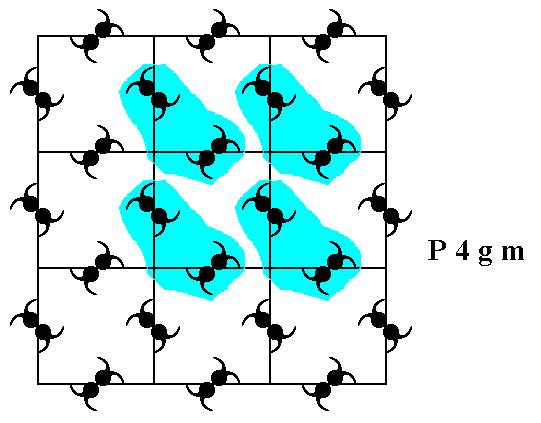Figure 4c.  Some areas in the pattern, representing Plane Group P4gm, are highligted in order to determine the actual motif that is being repeated throughout the structure.

```
```
Each highlighted area can be thought to be associated with a node of the net. So there should be much more such areas, each one of them associated with a node. In this way the nodes remain equivalent to each other, also when -- as in the present case -- motifs are inserted in the net.
The next Figure shows the four areas indicated above and their association with the nodes of the net.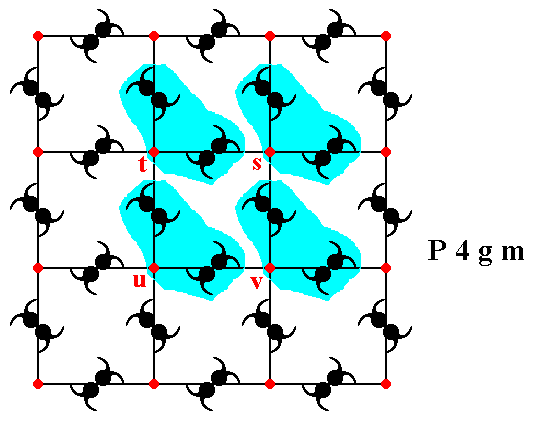Figure 4d.  The association of the four highlighted areas with the nodes (red) s, t, u, v of the square net. Each node must have such an associated area, in order to remain equivalent.

```
```
Figure 4e determines the actual motifs that are being repeated. These genuine motifs each consist of the motif s.str. (itself consisting of several motif units) and the motif s.l. (= the motif s.str. + its proper surroundings). One such motif is depicted.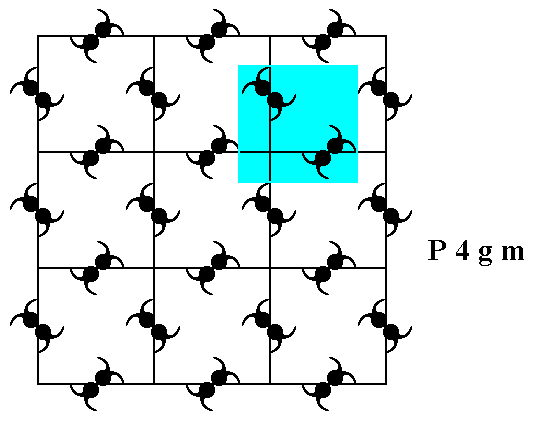Figure 4e.  A motif, when repeated along the vectors a and b (See Figure 4a), generates the periodic pattern of Figure 4.
The next Figure illustrates four such motifs.

```
```Figure 4f.  Four genuine equivalent motifs (highlighted with coloration), each associated with a lattice node.
Not only these four lattice nodes, but every node is in fact associated with such a motif. The symmetry of the motif is
m , i.e. it possesses one mirror line as its only symmetry element.

```
```
Let's consider the equivalence and non-equivalence of points (lattice nodes and other points) in the pattern representing the Plane Group P4gm. See Figure 4g.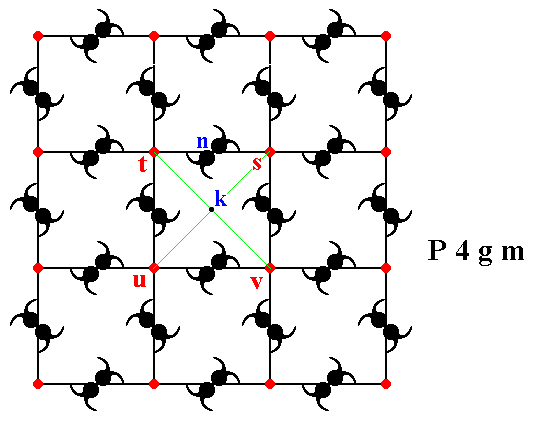Figure 4g.  The pattern representing the Plane Group P4gm. Some points (lattice nodes and other points) are indicated by letters.

```
```
If we interpret point s as a lattice point, then point t is equivalent to point s, and they are also equivalent to the points u and v. So s t u v can be chosen as the outline of the unit mesh.
Point n (situated precisely between the points t and s) is not equivalent to these points, because through point n does not go a 4-fold rotation axis (but a 2-fold rotation axis instead), while through point s indeed goes a 4-fold rotation axis, and also through the points t, u and v.
Point k also is not equivalent to point s, and also not to the points t, u and v, because the motif unitsat its left and right are oriented North-West---South-East, while they are oriented North-East---South-West left and right from point s, and the same goes for the points t, u and v. Consequently the unit mesh is not centered, the mesh is primitive (P).
```
```
We're now going to determine the total symmetry content of the pattern -- as depicted in Figure 4 -- representing the Plane Group P4gm.
To begin with, the next Figure shows that there is a 4-fold rotation axis at each lattice node. These axes are of course perpendicular to te plane of the drawing.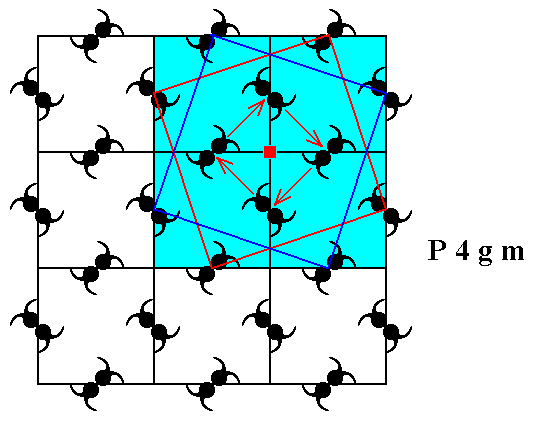Figure 4h.  There is a 4-fold rotation axis at every lattice node. One of them is shown.

```
```
The next Figure shows that there is also a 4-fold rotation axis at the center of each square defined by the lattice nodes. Also these axes are perpendicular to the plane of the drawing.Figure 4i.  The center of each square defined by the lattice nodes contains a 4-fold rotation axis. One is shown.

```
```
From Figures 4g and 4j it is clear that point n (lying exactly between points s and t) contains a 2-fold rotation axis, as well as any other point equivalent to n.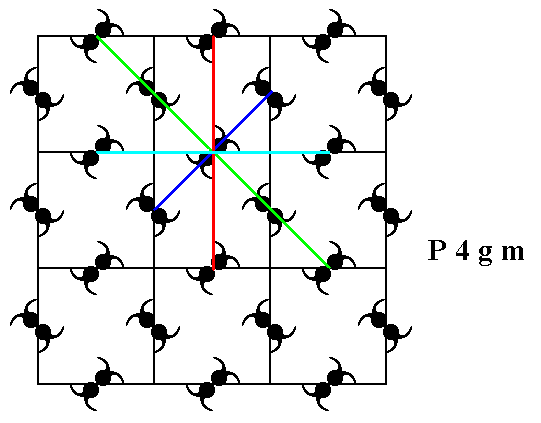Figure 4j.   A 2-fold rotation axis goes through point n (Figure 4g), as well as through any other point that is equivalent to n.

```
```
As further symmetry elements we can detect mirror lines. In the pattern representing Plane Group P4gm we can see one type of mirror line, namely diagonal mirror lines. Non-diagonal mirror lines are not supported by the pattern. See Figure 4k.Figure 4k.  The pattern representing Plane Group P4gm has diagonal mirror lines (m).
Four of them are depicted.

```
```
The pattern representing the Plane Group P4gm also has glide lines, diagonal and non-diagonal glide lines. See Figures 4l, 4m, 4n and 4o.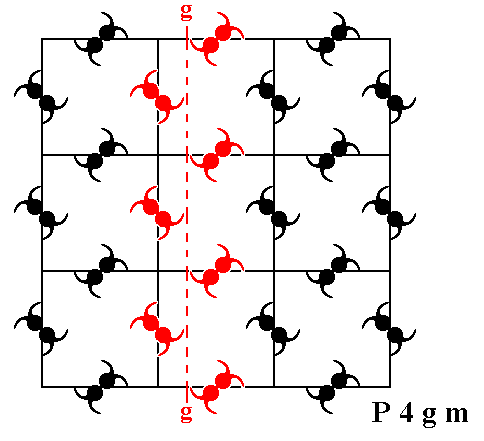Figure 4l.  The pattern representing Plane Group P4gm has non-diagonal glide lines (g). One of them is depicted (dashed line).

```
```Figure 4m.  The pattern representing Plane Group P4gm has further non-diagonal glide lines (g). One of them (perpendicular to the one depicted just above) is shown (dashed line).

```
```Figure 4n.  The pattern representing Plane Group P4gm has also diagonal glide lines (g). One of them (at 450 to the one depicted just above) is shown (dashed line).

```
```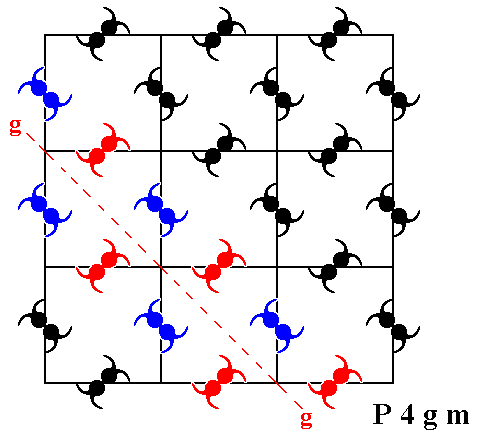Figure 4o.  The pattern representing Plane Group P4gm has further diagonal glide lines (g). One of them (perpendicular to the one depicted just above) is shown (dashed line).

```
```
In the next Figure we depict the total symmetry content of patterns representing the
Plane Group P4gm.Figure 4p.  Total symmetry content of the Plane Group P4gm.
Glide lines are indicated by red dashed lines.
Mirror lines are indicated by red solid lines.
4-fold rotation axes are indicated by small red solid squares.
2-fold rotation axes are indicated by small red solid ellipses.

```
```
So from the Square lattice we have now derived two Plane Groups, namely P4mm and P4gm, both associated with a point symmetry of 4mm.
Said in other words :   From the 2-D Tetragonal Crystal System we have considered its highest symmetric Class, 4mm, and this Class allows for two possible Plane Groups, namely P4mm and P4gm.

The Square Net can still accommodate for yet another motif, having a point symmetry 4, and representing yet another (and last) Class of the 2-D Tetragonal Crystal System. See Figure 5.Figure 5.  A periodic pattern of motifs based on a 2-D square lattice (net). This particular pattern represents the Plane Group P4. It must be imagined to extend indefinitely over the 2-D plane.

```
```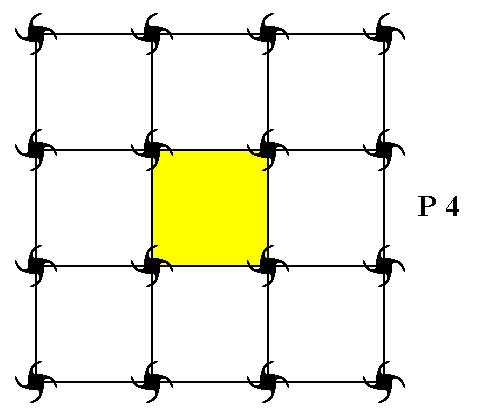Figure 5a.  A unit mesh choice (yellow) for the pattern representing the Plane Group P4. The point symmetry of this unit mesh is 4, and it is primitive, and as such denoted by the symbol P.

```
```
When we eliminate all translations we end up with a figure having 4 point symmetry. This then is the point symmetry associated with the pattern representing Plane Group P4 :Figure 5b.  Eliminating all translations yields the motif s.l.
Its point symmetry is
4.

```
```
As can be seen from Figure 5, the symmetry elements of the pattern representing Plane Group P4 are simple translations, 2-fold rotation axes and 4-fold rotation axes.
The next Figure shows the total symmetry content of the Plane Group P4.Figure 5c.  The total symmetry content of any pattern representing Plane Group P4. There are neither mirror lines, nor glide lines present.

```
```
Summarizing :   We have just derived another Plane Group, P4, from the Square Net (by inserting motifs). The point symmetry associated with this Group is 4.
So in considering the 2-D Tetragonal Crystal system (represented by the Square Net) we have found the Class 4. There is only one Plane Group associated with this Class, namely Plane Group P4.
```
```
In the next Part we will consider the 2-D Rectangular Crystal System. Two 2-D lattice types (nets) are possible there, a primitive net and a centered net. We will find two (2-D) Crystal Classes (planar Point Groups), belonging to the 2-D Rectangular Crystal System, and seven Plane Groups.
```

```
```